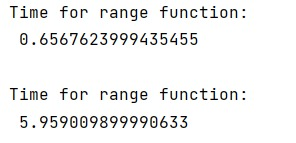# Built-in range() or numpy.arange(): which is more efficient?

Learn, which is more efficient method Python's built-in range() or NumPy's arange() method?
Submitted by Pranit Sharma, on January 17, 2023

NumPy is an abbreviated form of Numerical Python. It is used for different types of scientific operations in python. Numpy is a vast library in python which is used for almost every kind of scientific or mathematical operation. It is itself an array which is a collection of various methods and functions for processing the arrays.

## range() vs numpy.arange()

While iterating over a large array with a range expression, should we use Python's built-in range() function or numpy's arange() to get the best performance. The answer to this question depends upon the working of both methods.

For large arrays, a vectorised numpy operation is the fastest. If we want to loop, we should prefer xrange()/range() and avoid using numpy.arange().

In numpy we should use combinations of vectorized calculations, ufuncs and indexing to solve our problems as it runs at C speed. Looping over numpy arrays is inefficient compared to this.

Let us check the speed of both methods,

## Python code to understand - which is more efficient method Python's built-in range() or NumPy's arange() method

```import timeit

# For Loop using range
print("Time for range function:\n",timeit.Timer('for i in range(1):pass').timeit(),"\n")

import numpy as np

# For Loop using np.arange
print("Time for range function:\n",timeit.Timer('for i in np.arange(1):pass', globals=dict(np=np)).timeit(),"\n")
```

Output:What's New (MCQs)

Top Interview Coding Problems/Challenges!

IncludeHelp's Blogs

Languages: » C » C++ » C++ STL » Java » Data Structure » C#.Net » Android » Kotlin » SQL
Web Technologies: » PHP » Python » JavaScript » CSS » Ajax » Node.js » Web programming/HTML
Solved programs: » C » C++ » DS » Java » C#
Aptitude que. & ans.: » C » C++ » Java » DBMS
Interview que. & ans.: » C » Embedded C » Java » SEO » HR
CS Subjects: » CS Basics » O.S. » Networks » DBMS » Embedded Systems » Cloud Computing
» Machine learning » CS Organizations » Linux » DOS
More: » Articles » Puzzles » News/Updates